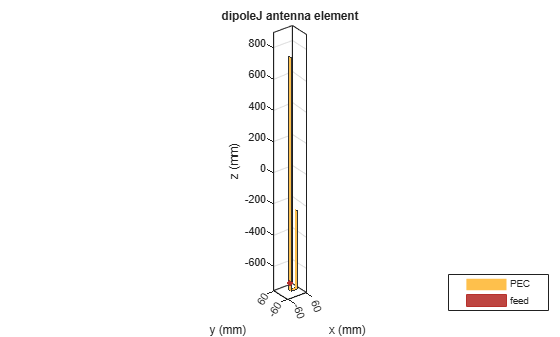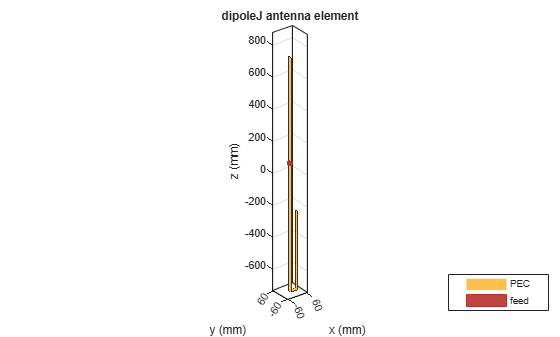# dipoleJ

Create J-dipole antenna

## Description

Use the `dipoleJ` object to create a J-dipole on the yz-plane. The antenna contains a half-wavelength radiator and a quarter-wavelength stub. The default antenna dimensions are set for an operating frequency of 144MHz.## Creation

### Syntax

``jdipole = dipoleJ``
``jdipole = dipoleJ(Name=Value)``

### Description

example

````jdipole = dipoleJ` creates a J-dipole antenna at an operating frequency of 144MHz.```

example

````jdipole = dipoleJ(Name=Value)` creates a J-dipole antenna with additional Properties specified by one or more name-value arguments. `Name` is the property name and `Value` is the corresponding value. You can specify several name-value pair arguments in any order as `Name1=Value1`, `...`, `NameN=ValueN`. Properties that you do not specify retain their default values.```

## Properties

expand all

Radiator length, specified as a positive scalar in meters.

Example: `0.9`

Data Types: `double`

Parallel line stub length, specified as a positive scalar in meters.

Example: `0.3`

Data Types: `double`

Strip width, specified as a positive scalar in meters.

Example: `0.0500`

Data Types: `double`

Space between the parallel line stub and the radiator, specified as a scalar in meters.

Example: `0.0500`

Data Types: `double`

Signed distance to the feed from the base of stub on the large arm, specified as a scalar in meters.

Example: `0.0345`

Data Types: `double`

Type of the metal used as a conductor, specified as a metal material object. You can choose any metal from the `MetalCatalog` or specify a metal of your choice. For more information, see `metal`. For more information on metal conductor meshing, see Meshing.

Example: `metal("Copper")`

Lumped elements added to the antenna feed, specified as a lumped element object. You can add a load anywhere on the surface of the antenna. By default, the load is at the origin. For more information, see `lumpedElement`.

Example: `lumpedElement(Impedance=75)`

Tilt angle of the antenna in degrees, specified as a scalar or vector. For more information, see Rotate Antennas and Arrays.

Example: `90`

Example: `Tilt=[90 90]`,`TiltAxis=[0 1 0;0 1 1]` tilts the antenna at 90 degrees about the two axes defined by the vectors.

Data Types: `double`

Tilt axis of the antenna, specified as one of these values:

• Three-element vector of Cartesian coordinates in meters. In this case, each coordinate in the vector starts at the origin and lies along the specified points on the x-, y-, and z-axes.

• Two points in space, specified as a 2-by-3 matrix corresponding to two three-element vectors of Cartesian coordinates. In this case, the antenna rotates around the line joining the two points.

• `"x"`, `"y"`, or `"z"` to describe a rotation about the x-, y-, or z-axis, respectively.

Example: `[0 1 0]`

Example: `[0 0 0;0 1 0]`

Example: `"Z"`

Data Types: `double` | `string`

## Object Functions

 `axialRatio` Axial ratio of antenna `beamwidth` Beamwidth of antenna `charge` Charge distribution on antenna or array surface `current` Current distribution on antenna or array surface `design` Design prototype antenna or arrays for resonance around specified frequency or create AI-based antenna from antenna catalog objects `efficiency` Radiation efficiency of antenna `EHfields` Electric and magnetic fields of antennas or embedded electric and magnetic fields of antenna element in arrays `impedance` Input impedance of antenna or scan impedance of array `info` Display information about antenna or array `mesh` Mesh properties of metal, dielectric antenna, or array structure `meshconfig` Change meshing mode of antenna, array, custom antenna, custom array, or custom geometry `optimize` Optimize antenna or array using SADEA optimizer `pattern` Plot radiation pattern and phase of antenna or array or embedded pattern of antenna element in array `patternAzimuth` Azimuth plane radiation pattern of antenna or array `patternElevation` Elevation plane radiation pattern of antenna or array `rcs` Calculate and plot radar cross section (RCS) of platform, antenna, or array `returnLoss` Return loss of antenna or scan return loss of array `show` Display antenna, array structures, shapes, or platform `sparameters` Calculate S-parameters for antennas and antenna arrays `vswr` Voltage standing wave ratio (VSWR) of antenna or array element

## Examples

collapse all

Create and view a default J-dipole antenna.

`d = dipoleJ`
```d = dipoleJ with properties: RadiatorLength: 0.9970 StubLength: 0.4997 Spacing: 0.0460 Width: 0.0200 FeedOffset: -0.6994 Conductor: [1x1 metal] Tilt: 0 TiltAxis: [1 0 0] Load: [1x1 lumpedElement] ```
`show(d)`Create and view a J-dipole antenna with the following specifications:

1. Radiator length = 0.978 m

2. Stub length = 0.485 m

3. FeedOffset = 0.049 m

```dj = dipoleJ(RadiatorLength=0.978, StubLength=0.485, ... FeedOffset=0.070); show(dj)```Calculate the impedance of the antenna over a frequency span 140MHz - 150MHz.

`impedance(dj,linspace(140e6,150e6,51));`## Version History

Introduced in R2018a